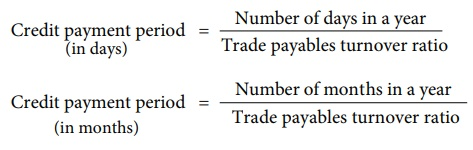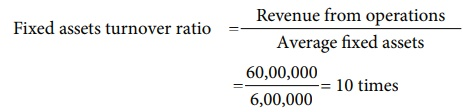Home | | Accountancy 12th Std | Turnover ratios

# Turnover ratios

Turnover ratios show how efficiently assets or other items have been used to generate revenue from operations. They are also called as activity ratios or efficiency ratios.

Turnover ratios

Turnover ratios show how efficiently assets or other items have been used to generate revenue from operations. They are also called as activity ratios or efficiency ratios. They show the speed of movement of various items. They are expressed as number of times in relation to the item compared.

The important turnover ratios are:

i.            Inventory turnover ratio

iv.            Fixed assets turnover ratio

## (i) Inventory turnover ratio

It indicates the number of times inventory is turned over to make revenue from operations (sales) during a particular accounting period. It is a comparison of cost of revenue from operations (cost of goods sold) with average amount of inventory during a given period. It is calculated as under:

Inventory turnover ratio = Cost of revenue from operations / Average inventoryCost of revenue from operations      = Purchases of stock in trade + Changes in inventories of finished goods + Direct expenses

(or)

= Revenue from operations – Gross profit

Tutorial note

Revenue from operations is the net sales.

Changes in inventory = Opening inventory – Closing inventory

Direct expenses = Wages + Carriage inwards + Freight inwards + Dock charges + Octroi + Import duty + Coal, gas, fuel and power + Other direct expenses

Average inventory = [ Opening inventory + Closing inventory ] / 2Cost of revenue from operations is taken because the inventory is always valued at cost except when net realisable value is lower than cost, it is valued at net realisable value. Greater the inventory turnover ratio, greater is the efficiency in the movement of stock. However, high inventory turnover ratio may also be due to insufficient inventory, buying in small quantities, etc. Similarly, a low inventory turnover ratio may be due to inclusion of obsolete items in inventory, etc. Hence, inventory turnover ratio must be analysed together with the related items.

Tutorial note

In the absence of opening inventory, closing inventory can be taken instead of average inventory.

### Inventory conversion period

Inventory conversion period is the time taken to sell the inventory. A shorter inventory conversion period indicates more efficiency in the management of inventory. It is computed as follows:Inventory conversion period  (in days) = Number of days in a year / Inventory turnover ratio

Inventory conversion period (in months) = Number of months in a year / Inventory turnover ratio

Illustration 8

From the given information calculate the inventory turnover ratio and inventory conversion period (in months) of Sania Ltd.SolutionCost of revenue from operations

= Opening inventory + Net Purchases + Direct expenses (carriage inwards) – Closing inventory

= 40,000 + 90,000 + 10,000 – 20,000

= ₹ 1,20,000## (ii) Trade receivables turnover ratio

Trade receivables turnover ratio is the comparison of credit revenue from operations with average trade receivables during an accounting period. It gives the velocity of collection of cash from trade receivables. It is calculated as follows:Trade receivables turnover ratio = Credit revenue from operations / Average trade receivables

Credit revenue from operations (net credit sales) is taken for trade receivables turnover ratio as trade receivables arise only from credit sales. Greater the trade receivables turnover ratio, greater is the efficiency of management in collection of receivables.

Tutorial note

Debt collection period

Debt collection period is the average time taken to collect the amount due from trade receivables. Lesser the debt collection period, greater is the efficiency of management in collection of cash from trade receivables. It is calculated as follows:

Debt collection period  (in days) = Number of days in a year / Trade receivables turnover ratio

Debt collection period  (in months) = Number of months in a year / Trade receivables turnover ratioIllustration 9

The credit revenue from operations of Harini Ltd. amounted to 9,60,000. Its debtors and bills receivable at the end of the accounting period amounted to 1,00,000 and 60,000 respectively. Calculate trade receivable turnover ratio and also collection period in months

Solution

Trade receivables turnover ratio = Credit revenue from operations / Average trade receivables

= 9,60,000 / 1,60,000 = 6 times.Trade receivables = Debtors + Bills receivable = 1,00,000 + 60,000 = ₹ 1,60,000

Tutorial note

Debt collection period = Number of months in a year / Trade receivables turnover ratio

= 12/6 = 2 months## (iii) Trade payables turnover ratio

Trade payables turnover ratio is the comparison of net credit purchases with average trade paybles during an accounting period. It gives the velocity of payment of cash towards trade payables. It is calculated as follows:

Trade payables turnover ratio = Net credit purchases / Average trade payables

Net credit purchases = Total credit purchases – Purchases returnsGreater the trade payable turnover ratio, better is the efficiency of the management in managing trade payable as it indicates that amount due to suppliers are settled quicker.

Tutorial note

Credit payment period

It is the average time taken by the business for payment of accounts payable. Lesser the credit payment period, greater is the efficiency of the management in managing accounts payable as it indicates quicker settlement of trade payables. It is calculated as follows:

Credit payment period  (in days) = Number of days in a year / Trade payables turnover ratio

Credit payment period  (in months) = Number of months in a year / Trade payables turnover ratioIllustration 10

From the following figures obtained from Kalpana Ltd, calculate the trade payables turnover ratio and credit payment period (in days).Solution## (iv) Fixed assets turnover ratio

Fixed assets turnover ratio gives the number of times the fixed assets are turned over during the year in relation to the revenue from operations. This ratio indicates the efficiency of utilisation of fixed assets.Fixed assets turnover ratio = Revenue from operations  / Average Fixed assets

Average fixed assets = [ Opening fixed assets + Closing fixed assets ] / 2

Greater the fixed assets turnover ratio better is the efficiency of management in utilisation of fixed assets.

Tutorial note

In the absence of opening fixed assets, closing fixed assets can be taken instead of average fixed assets.

Illustration 11

From the following information of Ashika Ltd., calculate fixed assets turnover ratio:

i.            Revenue from operations during the year were 60,00,000.

ii.            Fixed assets at the end of the year was 6,00,000.

Solution

Fixed assets turnover ratio = Revenue from operations / Average Fixed assets = 60,00,000 /6,00,000 = 10 timesTutorial note

As opening fixed assets are not given, fixed assets at the end are taken instead of average fixed assets.

Illustration 12

Calculate (i) Inventory turnover ratio (ii) Trade receivable turnover ratio (iii) Trade payable turnover ratio and (iv) Fixed assets turnover ratio from the following information obtained from Delphi Ltd.(i) Revenue from operations for the year 10,50,000

(ii) Purchases for the year 4,50,000

(iii) Cost of revenue from operations 6,00,000.

Assume that sales and purchases are for credit.

SolutionTags : Computation of ratios | Accountancy , 12th Accountancy : Chapter 9 : Ratio Analysis
Study Material, Lecturing Notes, Assignment, Reference, Wiki description explanation, brief detail
12th Accountancy : Chapter 9 : Ratio Analysis : Turnover ratios | Computation of ratios | Accountancy